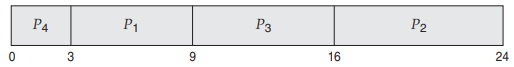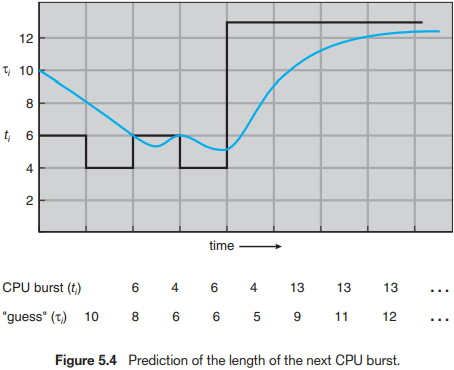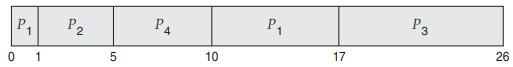#Shortest-Job-First CPU Scheduling AlgorithmA different approach to CPU scheduling is the shortest-job-firs (SJF) scheduling algorithm. This algorithm associates with each process the length of the process's next CPU burst. When the CPU is available, it is assigned to the process that has the smallest next CPU burst. If the next CPU bursts of two processes are the same, FCFS scheduling is used to break the tie. Note that a more appropriate term for this scheduling method would be the shortest-next-CPU-burst algorithm, because scheduling depends on the length of the next CPU burst of a process, rather than its total length. We use the term SJF because most people and textbooks use this term to refer to this type of scheduling.

As an example of SJF scheduling, consider the following set of processes, with the length of the CPU burst given in milliseconds:

 Process Burst Time P1 6 P2 8 P3 7 P4 3

Using SJF scheduling, we would schedule these processes according to the following Gantt chart:The waiting time is 3 milliseconds for process P1, 16 milliseconds for process P2, 9 milliseconds for process P3, and 0 milliseconds for process P4. Thus, the average waiting time is (3 + 16 + 9 + 0)/4 = 7 milliseconds. By comparison, if we were using the FCFS scheduling scheme, the average waiting time would be 10.25 milliseconds.

The SJF scheduling algorithm is probably optimal, in that it gives the minimum average waiting time for a given set of processes. Moving a short process before a long one decreases the waiting time of the short process more than it increases the waiting time of the long process. Consequently, the average waiting time decreases.

Although the SJF algorithm is optimal, it cannot be implemented at the level of CPU scheduling, as there is no way to know the length of the next CPU burst. One approach to this problem is to try to approximate SJF scheduling. We may not know the length of the next CPU burst, but we may be able to predict its value. We expect that the next CPU burst will be similar in length to the previous ones. By computing an approximation of the length of the next CPU burst, we can pick the process with the shortest predicted CPU burst.

The next CPU burst is generally predicted as an exponential average of the measured lengths of previous CPU bursts. We can define the exponential average with the following formula. Let tn be the length of the nth CPU burst, and let taun+1 be our predicted value for the next CPU burst. Then, for a, 0 <= a <= 1, define

taun+1 = atn + (1 - a)taun

The value of tn contains our most recent information, while taun stores the past history. The parameter a controls the relative weight of recent and past history in our prediction. If a = 0, then taun+1 = taun, and recent history has no effect (current conditions are assumed to be transient).

If a = 1, then taun+1 = taun, and only the most recent CPU burst matters (history is assumed to be old and irrelevant). More commonly, a = 1/2, so recent history and past history are equally weighted. The initial tau0 can be defined as a constant or as an overall system average. Figure 5.4 shows an exponential average with a = 1/2 and tau0 = 10.To understand the behavior of the exponential average, we can expand the formula for taun+1 by substituting for τn to find

taun+1 = atn + (1-a)atn-1 +...+ (1-a)jatn-j +...+ (1-a)n+1tau0

Typically, a is less than 1. As a result, (1 − a) is also less than 1, and each successive term has less weight than its predecessor.

The SJF algorithm can be either preemptive or nonpreemptive. The choice arises when a new process arrives at the ready queue while a previous process is still executing. The next CPU burst of the newly arrived process may be shorter than what is left of the currently executing process. A preemptive SJF algorithm will preempt the currently executing process, whereas a nonpreemptive SJF algorithm will allow the currently running process to finish its CPU burst. Preemptive SJF scheduling is sometimes called shortest-remainingtime-first scheduling.

As an example, consider the following four processes, with the length of the CPU burst given in milliseconds:

 Process Arrival Time Burst Time P1 0 8 P2 1 4 P3 2 9 P4 3 5

If the processes arrive at the ready queue at the times shown and need the indicated burst times, then the resulting preemptive SJF schedule is as depicted in the following Gantt chart:Process P1 is started at time 0, since it is the only process in the queue. Process P2 arrives at time 1. The remaining time for process P1 (7 milliseconds) is larger than the time required by process P2 (4 milliseconds), so process P1 is preempted, and process P2 is scheduled. The average waiting time for this example is [(10 − 1) + (1 − 1) + (17 − 2) + (5 − 3)]/4 = 26/4 = 6.5 milliseconds. Nonpreemptive SJF scheduling would result in an average waiting time of 7.75 milliseconds.

Other scheduling algorithms are: First-Come, First-Served; Priority; Round Robin; Multilevel Queue; Multilevel Feedback Queue. All are explained in Operating System Concepts.

Abraham Silberschatz is the Sidney J. Weinberg Professor of Computer Science at Yale University. Prior to joining Yale, he was the Vice President of the Information Sciences Research Center at Bell Laboratories. Prior to that, he held a chaired professorship in the Department of Computer Sciences at the University of Texas at Austin.

Professor Silberschatz is a Fellow of the Association of Computing Machinery (ACM), a Fellow of Institute of Electrical and Electronic Engineers (IEEE), a Fellow of the American Association for the Advancement of Science (AAAS), and a member of the Connecticut Academy of Science and Engineering.

Greg Gagne is chair of the Computer Science department at Westminster College in Salt Lake City where he has been teaching since 1990. In addition to teaching operating systems, he also teaches computer networks, parallel programming, and software engineering.